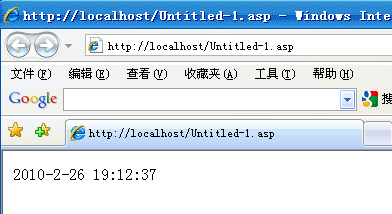# ASP环境下Now函数的应用

[日期：2010-02-26]   来源：IT部落窝  作者：IT部落窝   阅读：1315[字体： ]

Now函数：根据计算机系统设定的日期和时间返回当前的日期和时间值。

下面的程序示例利用 Now 函数返回当前的日期和时间：
<% =now() %>
运行代码如下：尝试着单击一下刷新按钮，这时会发现页面上显示的时间会随着单击而改变。

以上介绍的就是Now函数的基本用法。

同理，使用代码可以获得其它结果：
now() 获取当前系统日期和时间，ASP文件这样写：<%=now()%>
Year(now()) 获取年份， ASP文件这样写：<%=Year(now())%>
Month(now()) 获取当前月份，ASP文件这样写：<%=Month(now())%>
day(now()) 获取当天数，ASP文件这样写：<%=day(now())%>
Hour (now()) 获取小时数，ASP文件这样写：<%=hour(now())%>
Minute(now()) 获取分钟数，ASP文件这样写：<%=Minute(now())%>
Second(now()) 获取秒钟数，ASP文件这样写：<%=Second(now())%>
date() 获取当前系统日期，格式为：年-月-日，ASP文件这样写：<% =date() %>
time() 获取当前系统时间，格式为：时:分:秒，ASP文件这样写：<% =time() %>
Weekday 函数 ：返回代表一星期中某天的整数，ASP文件这样写：<%=weekdayname(weekday(now)) %>分享到： QQ空间 新浪微博 腾讯微博 人人网